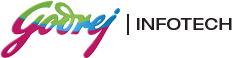# Placement Papers - GodrejGODREJ PAPER - 2006
Posted by :
Thoufiq Ahamed
(117)
Hi Friends

Pls go thru this paper. I am sure it will help u clear d apti. the very first page is full of instructions n rules(3)(veryimp) and examples(2) [read it carefully]

The test is known as box test 2D

10,5,7 in box1, box2, box 3. get 12 in box 1      U have to write the box no in the brackets

and 15 in box3.use add only.no of steps reqd =3.  along with d operation used.
box-1  box-2  box-3                       Result

Example 1: 10    5    7          box no.   box no.

Step1  5+7=12   5    7              +      =  

Step 2:   12     5   5+5=10           +      =  

Step 3:   12     5   10+5=15          +       =  

Rules:

1. n+m=n or m where n,m are box no\'s.(if in the final step u get it in this form..UR ANSWER IS RIGHT)n*m=n or m

2. don\'t remember..something related to subtraction I guess..

3. n/m=dividend..ignore the remainder
(if 9.9..take 9..donot round off)

Note:
U have to get the values in specified no. of steps given in each problem.if u get it in less no of steps then be sure U R GOING WRONG SOME where.n if u exceed the no of steps as mentioned in d problem then be ready for 25%negative marking (though they have given space for additional steps)

Moreover, even if u get d correct value in only one of the boxes.. ur score is zero for that particular problem. (consider the above example:if u get 12 in box 1 but 25 in box 3 then u will not gain ne mark..i.e,no marks for half right answer..no marks for d steps.). for each problem, they will mention the no.of steps as well as the operation .

Total questions: 10, Time:1 hr

1. 58,13,18 get 40 in box 1 and 85 in box3..use add only..3 steps.

2. 69,70,71  18,19,20  27,28,29  get -3,-4,-5 in box 2 ..use ne/all operations..4 steps

3. -1,0,2   -A    A(A-1)(A+1)   get A(A-1)(A-1)(A) in box 2 ..3 stepsuse multiply or divide

4. C  A   AorC   get C-A in box 1 and A-C in box 3 ...3 steps..use subtract only

5. AC  AB   BC    get 2C(B+A)-B(C-3A) in box1..(i.e3AB+2BC-BC+2AC)..3 steps..use add or subtract only

6. x or y , if x=7 y=12 ,   if x=19y=24   get even value in box 1 ..2 steps

7. p(price per dozen)    84(expise...)   7(no.of bats)   get total price+ expise in box1..4 steps..  use ne /all operations..4 steps

8. big statement problem ..similar to problem no7.

9. 17,42,3   get 50 in box 2 and 25 in box 3 ..3 steps..use add or subtract only.

10. I don\'t rem..

U have to be extremely quick wen solving these sums.1 hr seems long but tats not d case.some of the figures which I have written in this paper may get misplaced ..neways..best of luck to u all.

By, Arun